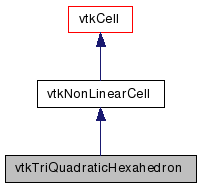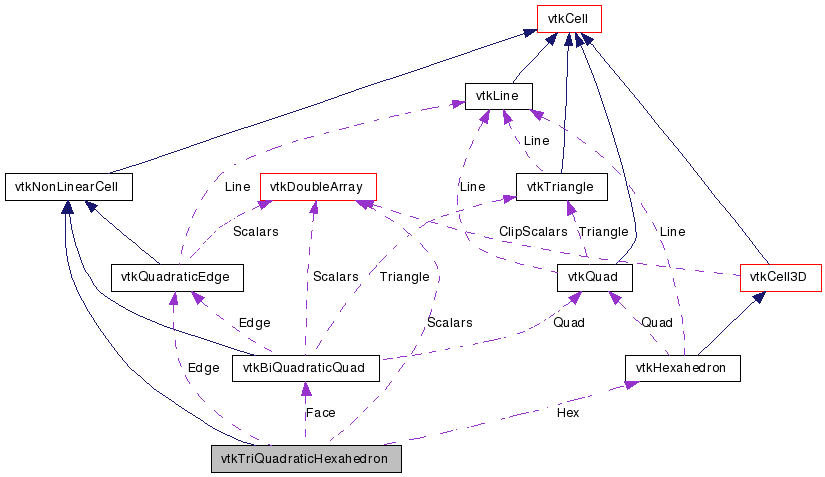`#include <vtkTriQuadraticHexahedron.h>`[legend][legend]

## Detailed Description

cell represents a parabolic, 27-node isoparametric hexahedron

vtkTriQuadraticHexahedron is a concrete implementation of vtkNonLinearCell to represent a three-dimensional, 27-node isoparametric triquadratic hexahedron. The interpolation is the standard finite element, triquadratic isoparametric shape function. The cell includes 8 edge nodes, 12 mid-edge nodes, 6 mid-face nodes and one mid-volume node. The ordering of the 27 points defining the cell is point ids (0-7,8-19, 20-25, 26) where point ids 0-7 are the eight corner vertices of the cube; followed by twelve midedge nodes (8-19); followed by 6 mid-face nodes (20-25) and the last node (26) is the mid-volume node. Note that these midedge nodes correspond lie on the edges defined by (0,1), (1,2), (2,3), (3,0), (4,5), (5,6), (6,7), (7,4), (0,4), (1,5), (2,6), (3,7). The mid-surface nodes lies on the faces defined by (first edge nodes id's, than mid-edge nodes id's): (0,1,5,4;8,17,12,16), (1,2,6,5;9,18,13,17), (2,3,7,6,10,19,14,18), (3,0,4,7;11,16,15,19), (0,1,2,3;8,9,10,11), (4,5,6,7;12,13,14,15). The last point lies in the center of the cell (0,1,2,3,4,5,6,7).

```
top
7--14--6
|      |
15  25  13
|      |
4--12--5

middle
19--23--18
|      |
20  26  21
|      |
16--22--17

bottom
3--10--2
|      |
11  24  9
|      |
0-- 8--1

```

Thanks:
Thanks to Soeren Gebbert who developed this class and integrated it into VTK 5.0.
Tests:

Definition at line 83 of file vtkTriQuadraticHexahedron.h.

static void InterpolationFunctions (double pcoords, double weights)
static void InterpolationDerivs (double pcoords, double derivs)
static int * GetEdgeArray (int edgeId)
static int * GetFaceArray (int faceId)
virtual void InterpolateFunctions (double pcoords, double weights)
virtual void InterpolateDerivs (double pcoords, double derivs)

## Public Types

typedef vtkNonLinearCell Superclass

## Public Member Functions

virtual const char * GetClassName ()
virtual int IsA (const char *type)
void PrintSelf (ostream &os, vtkIndent indent)
int CellBoundary (int subId, double pcoords, vtkIdList *pts)
void Contour (double value, vtkDataArray *cellScalars, vtkIncrementalPointLocator *locator, vtkCellArray *verts, vtkCellArray *lines, vtkCellArray *polys, vtkPointData *inPd, vtkPointData *outPd, vtkCellData *inCd, vtkIdType cellId, vtkCellData *outCd)
int EvaluatePosition (double x, double *closestPoint, int &subId, double pcoords, double &dist2, double *weights)
void EvaluateLocation (int &subId, double pcoords, double x, double *weights)
int Triangulate (int index, vtkIdList *ptIds, vtkPoints *pts)
void Derivatives (int subId, double pcoords, double *values, int dim, double *derivs)
virtual double * GetParametricCoords ()
void JacobianInverse (double pcoords, double **inverse, double derivs)
int GetCellType ()
int GetCellDimension ()
int GetNumberOfEdges ()
int GetNumberOfFaces ()
vtkCellGetEdge (int)
vtkCellGetFace (int)
void Clip (double value, vtkDataArray *cellScalars, vtkIncrementalPointLocator *locator, vtkCellArray *tetras, vtkPointData *inPd, vtkPointData *outPd, vtkCellData *inCd, vtkIdType cellId, vtkCellData *outCd, int insideOut)
int IntersectWithLine (double p1, double p2, double tol, double &t, double x, double pcoords, int &subId)

## Static Public Member Functions

static int IsTypeOf (const char *type)

## Protected Attributes

vtkHexahedronHex
vtkDoubleArrayScalars

## Member Typedef Documentation

Reimplemented from vtkNonLinearCell.

Definition at line 87 of file vtkTriQuadraticHexahedron.h.

## Constructor & Destructor Documentation

 vtkTriQuadraticHexahedron::vtkTriQuadraticHexahedron ( ) ` [protected]`

 vtkTriQuadraticHexahedron::~vtkTriQuadraticHexahedron ( ) ` [protected]`

## Member Function Documentation

 static vtkTriQuadraticHexahedron* vtkTriQuadraticHexahedron::New ( ) ` [static]`

Create an object with Debug turned off, modified time initialized to zero, and reference counting on.

Reimplemented from vtkObject.

 virtual const char* vtkTriQuadraticHexahedron::GetClassName ( ) ` [virtual]`

Reimplemented from vtkNonLinearCell.

 static int vtkTriQuadraticHexahedron::IsTypeOf ( const char * name ) ` [static]`

Return 1 if this class type is the same type of (or a subclass of) the named class. Returns 0 otherwise. This method works in combination with vtkTypeMacro found in vtkSetGet.h.

Reimplemented from vtkNonLinearCell.

 virtual int vtkTriQuadraticHexahedron::IsA ( const char * name ) ` [virtual]`

Return 1 if this class is the same type of (or a subclass of) the named class. Returns 0 otherwise. This method works in combination with vtkTypeMacro found in vtkSetGet.h.

Reimplemented from vtkNonLinearCell.

 static vtkTriQuadraticHexahedron* vtkTriQuadraticHexahedron::SafeDownCast ( vtkObject * o ) ` [static]`

Reimplemented from vtkNonLinearCell.

 void vtkTriQuadraticHexahedron::PrintSelf ( ostream & os, vtkIndent indent ) ` [virtual]`

Methods invoked by print to print information about the object including superclasses. Typically not called by the user (use Print() instead) but used in the hierarchical print process to combine the output of several classes.

Reimplemented from vtkNonLinearCell.

 int vtkTriQuadraticHexahedron::GetCellType ( ) ` [inline, virtual]`

Implement the vtkCell API. See the vtkCell API for descriptions of these methods.

Implements vtkCell.

Definition at line 93 of file vtkTriQuadraticHexahedron.h.

 int vtkTriQuadraticHexahedron::GetCellDimension ( ) ` [inline, virtual]`

Implement the vtkCell API. See the vtkCell API for descriptions of these methods.

Implements vtkCell.

Definition at line 94 of file vtkTriQuadraticHexahedron.h.

 int vtkTriQuadraticHexahedron::GetNumberOfEdges ( ) ` [inline, virtual]`

Implement the vtkCell API. See the vtkCell API for descriptions of these methods.

Implements vtkCell.

Definition at line 95 of file vtkTriQuadraticHexahedron.h.

 int vtkTriQuadraticHexahedron::GetNumberOfFaces ( ) ` [inline, virtual]`

Implement the vtkCell API. See the vtkCell API for descriptions of these methods.

Implements vtkCell.

Definition at line 96 of file vtkTriQuadraticHexahedron.h.

 vtkCell* vtkTriQuadraticHexahedron::GetEdge ( int ) ` [virtual]`

Implement the vtkCell API. See the vtkCell API for descriptions of these methods.

Implements vtkCell.

 vtkCell* vtkTriQuadraticHexahedron::GetFace ( int ) ` [virtual]`

Implement the vtkCell API. See the vtkCell API for descriptions of these methods.

Implements vtkCell.

 int vtkTriQuadraticHexahedron::CellBoundary ( int subId, double pcoords, vtkIdList * pts ) ` [virtual]`

Given parametric coordinates of a point, return the closest cell boundary, and whether the point is inside or outside of the cell. The cell boundary is defined by a list of points (pts) that specify a face (3D cell), edge (2D cell), or vertex (1D cell). If the return value of the method is != 0, then the point is inside the cell.

Implements vtkCell.

 void vtkTriQuadraticHexahedron::Contour ( double value, vtkDataArray * cellScalars, vtkIncrementalPointLocator * locator, vtkCellArray * verts, vtkCellArray * lines, vtkCellArray * polys, vtkPointData * inPd, vtkPointData * outPd, vtkCellData * inCd, vtkIdType cellId, vtkCellData * outCd ) ` [virtual]`

Generate contouring primitives. The scalar list cellScalars are scalar values at each cell point. The point locator is essentially a points list that merges points as they are inserted (i.e., prevents duplicates). Contouring primitives can be vertices, lines, or polygons. It is possible to interpolate point data along the edge by providing input and output point data - if outPd is NULL, then no interpolation is performed. Also, if the output cell data is non-NULL, the cell data from the contoured cell is passed to the generated contouring primitives. (Note: the CopyAllocate() method must be invoked on both the output cell and point data. The cellId refers to the cell from which the cell data is copied.)

Implements vtkCell.

 int vtkTriQuadraticHexahedron::EvaluatePosition ( double x, double * closestPoint, int & subId, double pcoords, double & dist2, double * weights ) ` [virtual]`

Given a point x return inside(=1), outside(=0) cell, or (-1) computational problem encountered; evaluate parametric coordinates, sub-cell id (!=0 only if cell is composite), distance squared of point x to cell (in particular, the sub-cell indicated), closest point on cell to x (unless closestPoint is null, in which case, the closest point and dist2 are not found), and interpolation weights in cell. (The number of weights is equal to the number of points defining the cell). Note: on rare occasions a -1 is returned from the method. This means that numerical error has occurred and all data returned from this method should be ignored. Also, inside/outside is determine parametrically. That is, a point is inside if it satisfies parametric limits. This can cause problems for cells of topological dimension 2 or less, since a point in 3D can project onto the cell within parametric limits but be "far" from the cell. Thus the value dist2 may be checked to determine true in/out.

Implements vtkCell.

 void vtkTriQuadraticHexahedron::EvaluateLocation ( int & subId, double pcoords, double x, double * weights ) ` [virtual]`

Determine global coordinate (x) from subId and parametric coordinates. Also returns interpolation weights. (The number of weights is equal to the number of points in the cell.)

Implements vtkCell.

 int vtkTriQuadraticHexahedron::Triangulate ( int index, vtkIdList * ptIds, vtkPoints * pts ) ` [virtual]`

Generate simplices of proper dimension. If cell is 3D, tetrahedron are generated; if 2D triangles; if 1D lines; if 0D points. The form of the output is a sequence of points, each n+1 points (where n is topological cell dimension) defining a simplex. The index is a parameter that controls which triangulation to use (if more than one is possible). If numerical degeneracy encountered, 0 is returned, otherwise 1 is returned. This method does not insert new points: all the points that define the simplices are the points that define the cell.

Implements vtkCell.

 void vtkTriQuadraticHexahedron::Derivatives ( int subId, double pcoords, double * values, int dim, double * derivs ) ` [virtual]`

Compute derivatives given cell subId and parametric coordinates. The values array is a series of data value(s) at the cell points. There is a one-to-one correspondence between cell point and data value(s). Dim is the number of data values per cell point. Derivs are derivatives in the x-y-z coordinate directions for each data value. Thus, if computing derivatives for a scalar function in a hexahedron, dim=1, 8 values are supplied, and 3 deriv values are returned (i.e., derivatives in x-y-z directions). On the other hand, if computing derivatives of velocity (vx,vy,vz) dim=3, 24 values are supplied ((vx,vy,vz)1, (vx,vy,vz)2, ....()8), and 9 deriv values are returned ((d(vx)/dx),(d(vx)/dy),(d(vx)/dz), (d(vy)/dx),(d(vy)/dy), (d(vy)/dz), (d(vz)/dx),(d(vz)/dy),(d(vz)/dz)).

Implements vtkCell.

 virtual double* vtkTriQuadraticHexahedron::GetParametricCoords ( ) ` [virtual]`

Return a contiguous array of parametric coordinates of the points defining this cell. In other words, (px,py,pz, px,py,pz, etc..) The coordinates are ordered consistent with the definition of the point ordering for the cell. This method returns a non-NULL pointer when the cell is a primary type (i.e., IsPrimaryCell() is true). Note that 3D parametric coordinates are returned no matter what the topological dimension of the cell.

Reimplemented from vtkCell.

 void vtkTriQuadraticHexahedron::Clip ( double value, vtkDataArray * cellScalars, vtkIncrementalPointLocator * locator, vtkCellArray * tetras, vtkPointData * inPd, vtkPointData * outPd, vtkCellData * inCd, vtkIdType cellId, vtkCellData * outCd, int insideOut ) ` [virtual]`

Clip this triquadratic hexahedron using scalar value provided. Like contouring, except that it cuts the hex to produce linear tetrahedron.

Implements vtkCell.

 int vtkTriQuadraticHexahedron::IntersectWithLine ( double p1, double p2, double tol, double & t, double x, double pcoords, int & subId ) ` [virtual]`

Line-edge intersection. Intersection has to occur within [0,1] parametric coordinates and with specified tolerance.

Implements vtkCell.

 static void vtkTriQuadraticHexahedron::InterpolationFunctions ( double pcoords, double weights ) ` [static]`

 static void vtkTriQuadraticHexahedron::InterpolationDerivs ( double pcoords, double derivs ) ` [static]`

 virtual void vtkTriQuadraticHexahedron::InterpolateFunctions ( double pcoords, double weights ) ` [inline, virtual]`

 virtual void vtkTriQuadraticHexahedron::InterpolateDerivs ( double pcoords, double derivs ) ` [inline, virtual]`

 static int* vtkTriQuadraticHexahedron::GetEdgeArray ( int edgeId ) ` [static]`

 static int* vtkTriQuadraticHexahedron::GetFaceArray ( int faceId ) ` [static]`

 void vtkTriQuadraticHexahedron::JacobianInverse ( double pcoords, double ** inverse, double derivs )

Given parametric coordinates compute inverse Jacobian transformation matrix. Returns 9 elements of 3x3 inverse Jacobian plus interpolation function derivatives.

## Member Data Documentation

 vtkQuadraticEdge* vtkTriQuadraticHexahedron::Edge` [protected]`

Definition at line 165 of file vtkTriQuadraticHexahedron.h.

 vtkBiQuadraticQuad* vtkTriQuadraticHexahedron::Face` [protected]`

Definition at line 166 of file vtkTriQuadraticHexahedron.h.

 vtkHexahedron* vtkTriQuadraticHexahedron::Hex` [protected]`

Definition at line 167 of file vtkTriQuadraticHexahedron.h.

 vtkDoubleArray* vtkTriQuadraticHexahedron::Scalars` [protected]`

Definition at line 168 of file vtkTriQuadraticHexahedron.h.

The documentation for this class was generated from the following file:

Generated on Mon Sep 27 18:57:30 2010 for VTK by1.5.6